# Lab 01: Introduction to Electrical Circuits

## Required Components List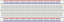Breadboard × 115 KΩ (brn grn orn gld) × 12.2 KΩ (red red red gld) × 1330 Ω (orn orn brn gld) × 1Green LED × 2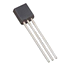2N4124 NPN Transistor × 1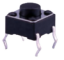Pushbutton Switch × 1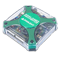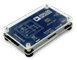Digilent Analog Discovery 2 (AD2)orAnalog Devices ADALM1000 (M1K) × 1

## Background

Electrical Circuits

Simply defined, an electric circuit is a closed-loop where through which charges (electrons) can continually flow. Electric circuits can be analog or digital.

• Analog circuits operate on continuous signals.
• Digital circuits operate on discrete, usually binary, signals.
Binary signals have two discrete values or logic levels:
• Logic 1: a high value of voltage (5V) in this lab
• Logic 0: a low value (0V or ground)

Fundamental components in analog circuits are Resistors, capacitors, inductors, diodes, transistors, and operational amplifiers. Fundamental components in digital circuits are transistors, logic gates, and integrated circuits (ICs). The ICs are a combination of logic gates that are integrated to implement simple digital circuits like counters and decoders and more complex digital systems like microprocessors and microcontrollers. As you'll discover in this experiment, all the digital components are actually analog circuits underneath.

Many circuits will have both analog and digital components such as in embedded systems which may have analog inputs such as sensors and analog outputs such as output voltages that control motor speed and a digital microcontroller programmed to control the system.

In this experiment, you will be introduced to the basics of voltage, current, and resistance which are the building blocks of any electric circuit. You will also discover how transistors work as electrical switches and see how they can be combined to build digital components known as logic gates.

Ohm's Law

Electricity is the movement and interaction of electrons which are charged particles. Voltage is a measure of the potential difference in charge between two points in an electric field. Voltage is measured in volts (V) which is a measure of the energy per unit charge. So a battery that is a source of energy (electrons) will have a potential difference in charge between its two terminals. For example, a 9V battery would supply 9 Joules of electric potential energy per every 1 Coulomb of charge which moves between its negative and positive terminals. Current, measured in amperes (A), is the rate at which electric charge flows past a point in a circuit. When a load is connected to the positive and negative terminals of a battery, an electric circuit will be formed and current will flow within the battery from its negative terminal to its positive terminal, then through the load and back to the negative terminal. A load in a circuit is an electrical component containing resistance, which dissipates energy as heat; examples are the appliances and lights in your home. Resistance is measured in Ohms (Ω). Ohm's Law defines the relationship between voltage, current, and resistance.

$V = IR$

Here $V$ represents the voltage, $I$ represents the current, and $R$ represents the resistance.

While all electrical components, including wires, have some level of resistance, Resistors are manufactured components with specific ohmic values designed to control the amount of current flowing through a circuit. They are passive components that consume power. Resistors have two terminals (end-points) and are represented by a zig-zag line such as: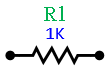Here R1 refers to a specific resistor in a circuit, and 1K is an abbreviation of 1 KΩ (or 1x103 Ohms) which is the value of the resistor. Resistors are manufactured to provide various values of resistance and, as mentioned earlier, are color-coded to indicate those values.

## Experiments

Every experiment section that requires you to build a circuit and test it has an asterisk (*). For those sections:

• For the in-class lab: Demonstrate the working circuit to your lab instructor.
• For online lab: Take a video to describe your circuit, upload the video to YouTube, and put the link in the report.